+

# Weighted Average Method

Author: Sophia Tutorial
##### Description:

Calculate the ending inventory and cost of goods sold using the weighted average method.

(more)### Developing Effective Teams

*No strings attached. This college course is 100% free and is worth 1 semester credit.

37 Sophia partners guarantee credit transfer.

299 Institutions have accepted or given pre-approval for credit transfer.

* The American Council on Education's College Credit Recommendation Service (ACE Credit®) has evaluated and recommended college credit for 32 of Sophia’s online courses. Many different colleges and universities consider ACE CREDIT recommendations in determining the applicability to their course and degree programs.

Tutorial
what's covered
This tutorial will cover the inventory cost flow assumption known as the weighted average method.

Our discussion breaks down as follows:

1. Weighted Average Method
2. Weighted Average Method and Cost of Goods Sold

## 1. Weighted Average Method

The weighted average method is an inventory valuation method based on average cost per unit. It helps to provide information about cost of goods sold and ending inventory based on average cost per unit.

The weighted average method is based on total cost and total units. The total cost of inventory units available for sale is divided by the total units that are available for sale, to provide the average cost per unit.

For the weighted average method, there is no concern for the timing of inventory purchases. It doesn't matter if inventory was purchased at the beginning of the period or at the end of the period--it's all based on the average cost per unit.

The argument for using the weighted average method is that inventory is very complex and it can be difficult to determine which exact units you're selling. Are you selling the units you purchased at the beginning of the period, or are you selling the units purchased at the end of the period? As you can see, it can be difficult to monitor and measure the exact flow of your inventory.

## 2. Weighted Average Method and Cost of Goods Sold

Before we discuss the weighted average method and cost of goods sold, let's revisit the cost of goods sold calculation. We start with beginning inventory and add cost of goods purchased to give us goods available for sale. Then, we subtract out ending inventory to equal cost of goods sold.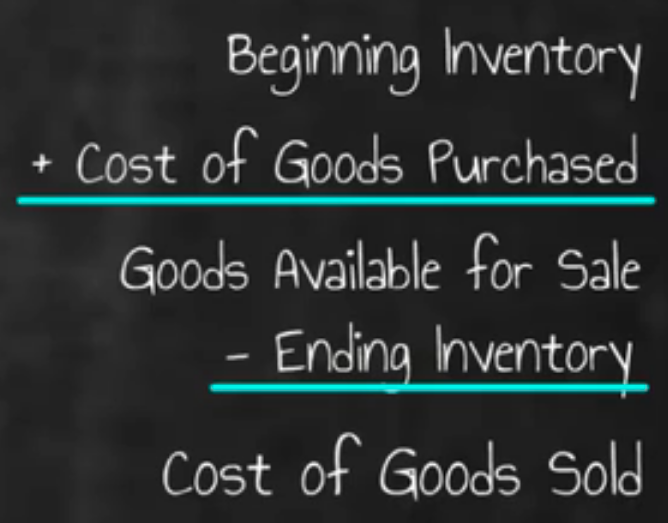Let's walk through an example of calculating cost of goods sold using the inventory valuation method of FIFO. Below you will see a spreadsheet outlining the cost of goods sold calculation. We will begin with the first line, beginning inventory. Beginning inventory is pulled from our balance sheet or from our trial balance. You can also see there is a schedule, detailing beginning inventory and purchases.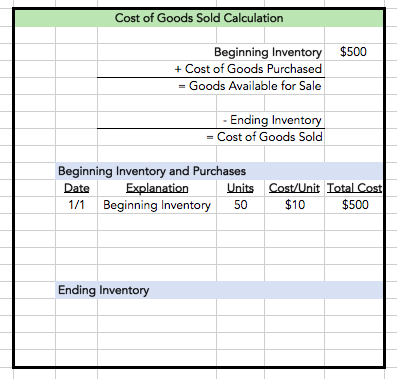Once we have plugged in beginning inventory, we need to determine cost of goods purchased. If you look at the detail of all the purchases made, you can see that we made three purchases throughout the year.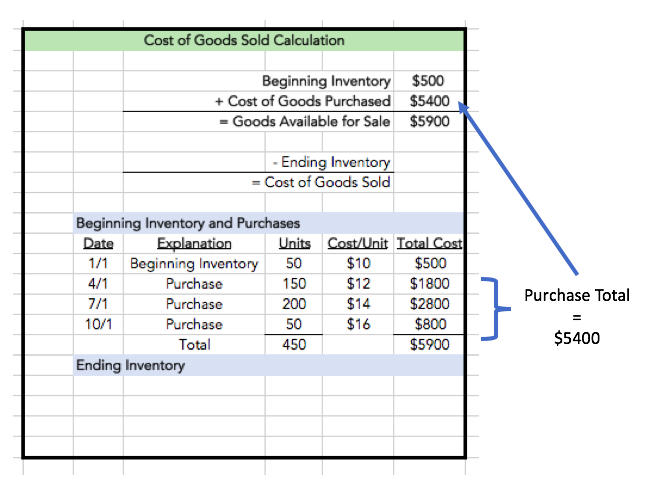We can take the total of those purchases, which is \$5,400, and drop that into the schedule, which means we now have total goods available for sale of \$5,900. Note that this is the total of beginning inventory plus all of the purchases.

Now we need to determine our ending inventory. So, how do we calculate our ending inventory in the weighted average method? Well, we need to know the average cost per unit. Therefore, we take our total cost of \$5,900 and divide by the 450 total units to calculate the average cost per unit.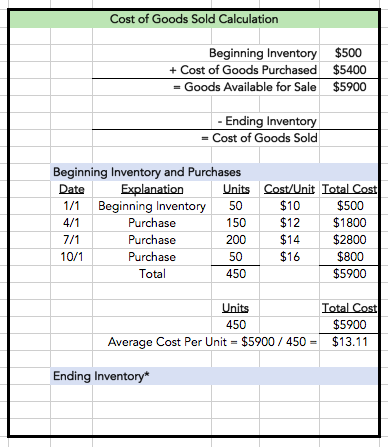The average cost per unit is \$13.11.

Now, if you look at the ending inventory schedule below, we're going to make the assumption that we have 100 units left in our ending inventory. The next calculation, then, is to take those 100 units and multiply them by our average cost per unit (\$13.11) to get the total cost of our ending inventory, which is \$1,311.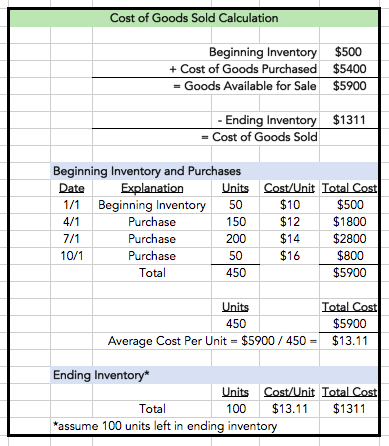Then, we take that ending inventory number and drop it into the cost of goods sold calculation, and subtract it from our goods available for sale to give us our cost of goods sold, which in this case is \$4,589.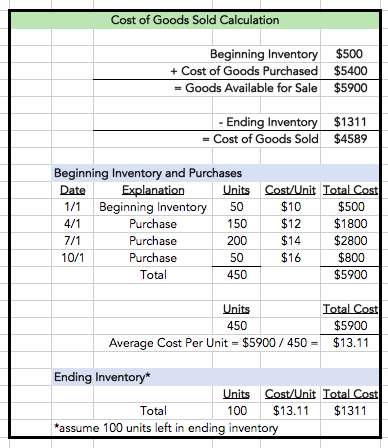summary
Today we learned about an inventory valuation method known as the weighted average method. The weighted average method is based on dividing total cost by total units to provide an average cost per unit. We also performed a calculation of cost of goods sold using the weighted average method.

Source: Adapted from Sophia instructor Evan McLaughlin.

Rating